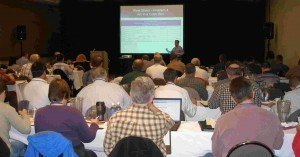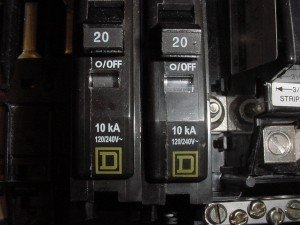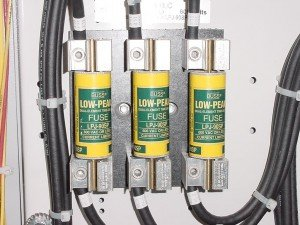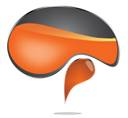## Short Circuit Analysis

Course No.: SC101
CEUs 0.8

### OverviewThis course was developed and is taught by Jim Phillips, P.E. Learn how to perform short circuit analysis / calculations and equipment adequacy evaluations. Understand the importance of X/R ratios, symmetrical vs. asymmetrical faults and how series ratings work. Many calculation examples are used to illustrate how to perform an analysis. The methods are based on the IEEE Red and Violet books. This class can be paired with the Protective Device Coordination Class for 2 days of training. This class is loaded with many in class examples and problems for a hands on learning experience.

Jim has developed this course based on over 30 years of extensive experience with industrial, commercial and utility power systems. Even instructors from other training companies have attended Jim’s classes to see how it’s done. You will learn how to conduct a short circuit study.

Training manual
Jim’s short circuit calculation worksheets
Many calculation examples and problems
8 hours of Continuing Education Credit

### Have This Class On-Site at Your Location

You can also have this class conducted on-site at your location.  Contact our Program Director at 800.874.8883 to see about having Jim teach this class to your staff at your company’s facilities.  Contact us for your custom on-site training proposal.

### SHORT CIRCUIT ANALYSISSHORT CIRCUIT ANALYSIS – INTRODUCTION
Short Circuit Study Requirements, NEC® 110.9 and 110.10, Interrupting and Withstand Ratings, Data Requirements, Available Utility Short Circuit Current, Conductor Impedance, Source Impedance, X/R Ratio, Per Phase Calculations, Thevenin Equivalent, Impedance

CONDUCTOR IMPEDANCE AND SHORT CIRCUIT CALCULATIONS
Determining the Source Impedance, Calculating the Conductor Impedance, Conductor Impedance Tables, Conductor Calculation Worksheets.  In Class Problems – Short Circuit Calculations with Conductor Impedance

TRANSFORMER IMPEDANCE AND SHORT CIRCUIT CALCULATIONS
Transformer Testing and Percent Impedance, X/R Ratio,  Using Percent Impedance for Short Circuit Calculations,  Determining the Source Impedance in Percent, Infinite Bus Calculations, Transformer Calculation Worksheets.  In Class Problems – Short Circuit Calculations with Transformer Impedance

MOTOR CONTRIBUTION
Theory of Motor Short Circuit Contribution, Sub-Transient Reactance, Xd”, Effect of Motor Contribution on Short Circuit Current, Multipliers for Motor Contribution.  In Class Problems – Consideration of Motor Contribution

DEVICE INTERRUPTING RATINGS
Circuit Breaker and Fuse Interrupting Ratings, UL and ANSI Testing Methods, Symmetrical and Asymmetrical Short Circuit Current, Effect of X/R Ratio on Interrupting Ratings, Multiplying Factors when the X/R and Asymmetry are Too Large

SERIES RATINGS
Development of Series Ratings, Proper Application of Series Ratings, Dynamic Impedance, Fully Rated vs. Series Rated, Current Limitation, Let Thru Current, U.L. Tests

CASE PROBLEM
Short Circuit Study of Small Industrial System.  Calculations Include Source, Conductor and Transformer Impedance, Motor Contribution and Protective Device Adequacy Evaluation

Attend This Class to See How To:Perform Short Circuit Calculations
Understand the Importance of Utility Data
Understand why is the X/R ratio Important with Asymmetry
Correctly Develop Series Ratings
Calculate Short Circuit Motor Contribution
Understand a Transformer’s Importance with Short Circuit Current

What questions do I ask the utility company?
What is the X/R ratio?
How does the X/R ratio effect a device’s interrupting rating?
What is motor contribution?
How do I calculate motor contribution on new systems with an undefined load?
• Is a short circuit study legally required?
What kind of data is required for the short circuit studies?
What if I can’t find all of the data, what assumptions can I make?
Why is the L/E ratio tm so important?
• How do current limiting fuses operate?
What is a symmetrical current vs. asymmetrical current?
How do I properly apply series ratings?

Questions?For questions, registration information or to discuss holding this class at your location as an on-site training program, contact our Program Director at 800.874.8883

Brainfiller, Inc. | P.O. Box 12024 | Scottsdale, AZ 85267